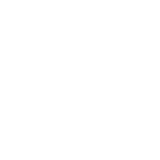Categories

# Algorithms and Flowcharts

## Objective Type Questions

### Fill in the blanks with the correct words:

1. A flowchart is a diagrammatic representation of a program.
2. The process box is rectangular in shape.
3. The decision box has two output lines; one for True and other for False.
4. A parallelogram represents input/output in a flowchart.
5. The flowchart symbols are connected by flow lines.

### State whether True or False and also correct the false statement(s):

1. The decision box is circular in shape. (FALSE)
Diamond in shape.
2. There should be only one start box in a flowchart. (TRUE)
3. A flowchart must be drawn on a single page. (FALSE)
Can be drawn on multiple pages.
4. You can use connectors in a  flowchart. (TRUE)
5. An algorithm is a pictorial form of a flowchart. (FALSE)
Flowchart is a pictorial form of a flowchart.

### Choose the correct option:

1. The symbol of start/stop is a/an:
a. Rectangle
b. Rhombus
c. Parallelogram
d. Oval
2. It has one incoming and two outgoing flow lines.
a. Input/Output box
b. Process box
c. Decision box
d. Start box
3. It indicates the flow of information or sequence of events.
a. Input/Output
b. Processing
c. Decision
d. Flow line
4. Which symbol represents Input/Output in a flowchart?
a. Rectangle
b. Parallelogram
c. Oval
d. Decision
5. What is the use of the oval symbol in a flowchart?
a. Used to show the start of a program only.
b. Used to show the end of a program only.
c. Used to show the starting and ending of a program.
d. None of these

## Descriptive Type Questions

1. What is the difference between an Algorithm and a Flowchart?
An algorithm is a finite set of step-wise instructions that should be followed to perform a specific task like solving a logical or a mathematical problem.
A flowchart is a diagrammatic representation of a problem-solving process in which steps are laid out in logical order.
2. What are the rules for flowcharting?
Following are the rules for flowcharting:
a. A flowchart should have only one Start and one Stop symbol.
b. The general direction of flow in a flowchart is from top to bottom or from left to right.
c. Arrowheads are used to indicate the flow of information or sequence of steps.
d. The arrow lines should not cross each other.
e. Off-page and on-page connectors should be used when joining parts of a flowchart spanning multiple pages/same page.
3. Explain the different symbols used in a flowchart.
Following are the different symbols used in a flowchart:
a. Oval (Start/Stop box): Used to indicate the start and end of the program.
b. Rectangle (Process box): Indicates processes or actions.
c. Parallelogram (Input/Output box): Indicates input and output of a program.
d. Diamond (Decision box): Used in situation which demands one to choose either ‘Yes’ or ‘No’.
e. Arrow line (Flow lines): Shows the flow of logic in a flowchart.
4. What are the two types of connectors in a flowchart? Explain their uses.
Following are the two types of connectors in a flowchart:
a. Off-page connectors: Used for joining the parts of a flowchart that occupies more than one page.
b. On-page connectors: To join the parts of a flowchart contained within one page.
5. What are the characteristics of a good algorithm?
Following are the characteristics of a good algorithm: